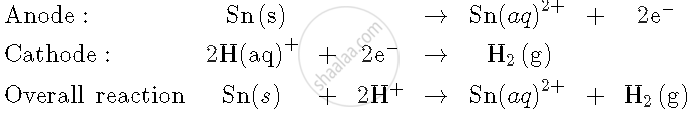Share

# Write the Cell Reaction and Calculate the E.M.F of the Following Cell at 298 K: Sn(S) | Sn^(2+) (0.004 M) || H^(+) (0.020 M) | H_2 (G) ("1 Bar") | Pt(S) - Chemistry

#### Question

Write the cell reaction and calculate the e.m.f of the following cell at 298 K:

Sn(s) | Sn^(2+) (0.004 M) || H^(+) (0.020 M) | H_2 (g) ("1 bar") | Pt(s)

(Given: E_(Sn^(2+)"/"Sn)^0 = -0.14 V)

#### SolutionE_"cell"^0 = E_(Sn"/"Sn^(+2))- 0.0

= -(-0.14)

= 0.14 V

E_"cell  = E_(cell)^0 - 0.059/2 log  ([H_2][Sn^(+2)])/[H^(+)]^2

= 0.14 - 0.059/2  log  ((1)(0.004))/(0.02)^2

= 0.14 - 0.059/2 log (10)

= 0.14 - 0.0295

= +0.1105 V

Is there an error in this question or solution?
Write the Cell Reaction and Calculate the E.M.F of the Following Cell at 298 K: Sn(S) | Sn^(2+) (0.004 M) || H^(+) (0.020 M) | H_2 (G) ("1 Bar") | Pt(S) Concept: Galvanic Cells - Measurement of Electrode Potential.